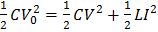# A capacitor of capacity 2is charged to a potential difference of 12 V. It is then connected across an inductor of inductance. What is the current (in A) in the circuit at a time when the potential difference across the capacitor is 6.0 V?

## Question ID - 154100 :- A capacitor of capacity 2is charged to a potential difference of 12 V. It is then connected across an inductor of inductance. What is the current (in A) in the circuit at a time when the potential difference across the capacitor is 6.0 V?

3537

 (6) From energy conservation:In the given circuit, initially switchis closed, andandare open. After charging of capacitor, atis opened andandare closed. If the relation between inductance, capacitance and resistance is, then find the time (in s) after which current passing through capacitor and inductor will be same (given)